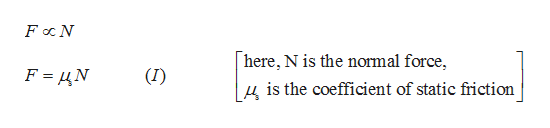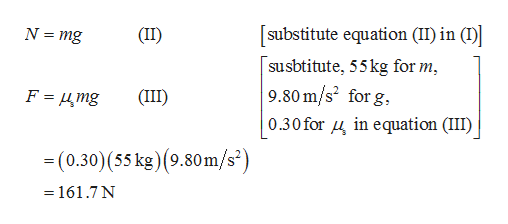# A 55-kg box rests on a horizontal surface. The coefficient of static friction between the box the surface is 0.30, and the coefficient of kinetic friction is 0.20. What horizontal force must be applied to the box to cause it to start sliding along the surface?

Question
3114 views

A 55-kg box rests on a horizontal surface. The coefficient of static friction between the box the surface is 0.30, and the coefficient of kinetic friction is 0.20. What horizontal force must be applied to the box to cause it to start sliding along the surface?

check_circle

Step 1

The mass of the box=55kg.

The coefficient of static friction =0.30.

The coefficient of kinetic friction=0.20.

To make the object just slide along the surface from rest it is required to overcome the static friction.

The force should be applied to the box is directly proportional to the normal force.help_outlineImage TranscriptioncloseFoc N here, N is the normal force, F N (I) |4 is the coefficient of static friction fullscreen
Step 2

Since the box is at rest the normal force wi...help_outlineImage Transcriptionclosesubstitute equation (II) in (I) N mg (II) susbtitute, 55kg for m 9.80 m/s2 for g, 0.30 for 4inequation (III) F = 4mg (II) -(0.30) (55 kg) (9.80m/s) =161.7 N fullscreen

### Want to see the full answer?

See Solution

#### Want to see this answer and more?

Solutions are written by subject experts who are available 24/7. Questions are typically answered within 1 hour.*

See Solution
*Response times may vary by subject and question.
Tagged in

### Kinematics